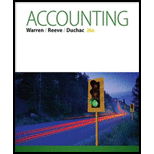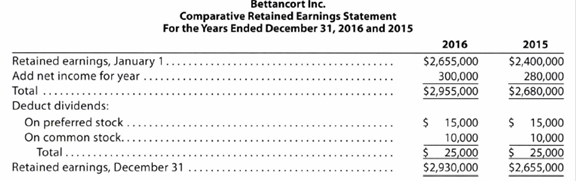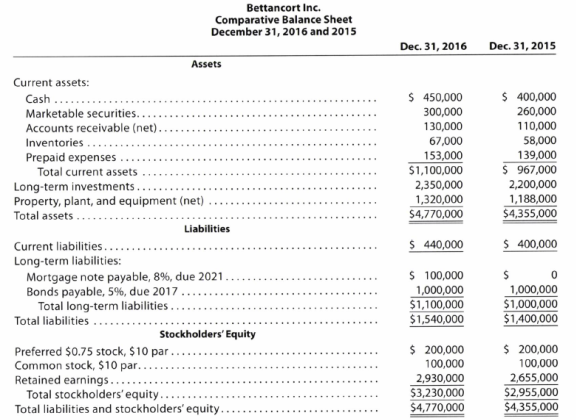# Nineteen measures of solvency and profitability The comparative financial statements of Bettancort Inc. are as follows. The market price of Bettancort Inc. common stock was $71.25 on December 31, 2016. Instructions Determine the following measures for 2016, rounding to one decimal place: 1. Working capital 2. Current ratio 3. Quick ratio 4. Accounts receivable turnover 5. Number of days’ sales in receivables 6. Inventor) turnover 7. Number of days’ sales in inventory 8. Ratio of fixed assets to long-term liabilities 9. Ratio of liabilities to stockholders’ equity 10. Number of times interest charges are earned 11. Number of times preferred dividends are earned 12. Ratio of sales to assets 13. Rate earned on total assets 14. Rate earned on stockholders’ equity 15. Rate earned on common stockholders’ equity 16. Earnings per share on common stock 17. Price-earnings ratio 18. Dividends per share of common stock 19. Dividend yieldBuyFindarrow_forward ### Accounting (Text Only) 26th Edition Carl Warren + 2 others Publisher: Cengage Learning ISBN: 9781285743615 #### Solutions Chapter SectionBuyFindarrow_forward ### Accounting (Text Only) 26th Edition Carl Warren + 2 others Publisher: Cengage Learning ISBN: 9781285743615 Chapter 17, Problem 17.4APR Textbook Problem 4 views ## Nineteen measures of solvency and profitabilityThe comparative financial statements of Bettancort Inc. are as follows. The market price of Bettancort Inc. common stock was$71.25 on December 31, 2016.InstructionsDetermine the following measures for 2016, rounding to one decimal place:1. Working capital2. Current ratio3. Quick ratio4. Accounts receivable turnover5. Number of days’ sales in receivables6. Inventor) turnover7. Number of days’ sales in inventory8. Ratio of fixed assets to long-term liabilities9. Ratio of liabilities to stockholders’ equity10.    Number of times interest charges are earned11. Number of times preferred dividends are earned12.     Ratio of sales to assets13. Rate earned on total assets14.     Rate earned on stockholders’ equity15.     Rate earned on common stockholders’ equity16.     Earnings per share on common stock17.     Price-earnings ratio18.     Dividends per share of common stock19.     Dividend yield

To determine

Financial Ratios: Financial ratios are the metrics used to evaluate the capabilities, profitability, and overall performance of a company.

The following ratios measures for 2016.

1. 1. Working capital
2. 2. Current ratio
3. 3. Quick ratio
4. 4. Accounts receivable turnover
5. 5. Number of days' sales in receivables
6. 6. Inventory turnover ratio
7. 7. Number of days' sales in inventory
8. 8. Ratio of fixed assets to long-term liabilities
9. 9. Ratio of liabilities to stockholders’ equity
10. 10. Number of times interest charges are earned
11. 11. Number of times preferred dividends are earned
12. 12. Ratio of sales to assets
13. 13. Rate earned on total assets
14. 14. Rate earned on stockholders' equity
15. 15. Return on common stockholders' equity
16. 16. Earnings per share on common stock
17. 17. Price earnings ratio
18. 18. Dividends per share of common stock
19. 19. Dividend yield

### Explanation of Solution

1.  Working capital

Working capital  = Current assets – Current liabilities = $1,100,000 –$440,000= $660,000 Note: Working capital is determined as the difference between current assets and current liabilities. Formula: Working capital = Current assets – Current liabilities 2. Current ratio Current ratio=Current assetsCurrentliabilities=$1,100,000$440,000=2.5 Note: Current ratio is used to determine the relationship between current assets and current liabilities. The ideal current ratio is 2:1. Current assets include cash and cash equivalents, short-term investments, net, accounts and notes receivables, net, inventories, and prepaid expenses and other current assets. Current liabilities include short-term obligations and accounts payable. Formula: Current ratio=Current assetsCurrentliabilities 3. Quick ratio Quick ratio =Quick assets Currentliabilities=$880,000$440,000=2.0 Note: Acid-Test Ratio is the ratio denotes that this ratio is a more rigorous test of solvency than the current ratio. It is determined by dividing quick assets and current liabilities. The acceptable acid-test ratio is 0.90 to 1.00. It is referred as quick ratio. Use the following formula to determine the acid-test ratio: Acid Ratio=Quick assetsCurrentliabilities 4. Accounts receivable turnover Accounts receivables turnover ratio}=Net credit salesAverage accounts receivables=$1,200,000$120,000=10.0 Note: Accounts receivables turnover ratio is mainly used to evaluate the collection process efficiency. It helps the company to know the number of times the accounts receivable is collected in a particular time period. Main purpose of accounts receivable turnover ratio is to manage the working capital of the company. This ratio is determined by dividing credit sales and sales return. Formula: Accounts receivables turnover ratio}=Net credit salesAverage accounts receivables Average accounts receivable is determined as follows: Average accounts receivables }(Opening accounts receivables + Closing accounts receivables )2=$130,000+$110,0002=$120,000

5. Number of days’ sales in receivables

Number of days’ sales in receivable }=Average accounts receivable Average daily sales=$120,000$3287.67=36.5days

Note:

Number of days’ sales in receivables is used to determine the number of days a particular company takes to collect accounts receivables.

Formula:

Number of days’ sales in receivable=Average accounts receivable Average daily sales

Average daily sales are determined by dividing sales by 365 days.

Average daily sales = Sales365days=$1,200,000365days=$3,287.67

6. Inventory turnover ratio

Inventory turnover ratio =Cost of goods soldAverage inventory=$500,000$62,500=8.0times

Note:

Inventory turnover ratio is used to determine the number of times inventory used or sold during the particular accounting period.

Formula:

Inventory turnover=Cost of goods soldAverage inventory

Average inventory is determined as below:

Average inventory = (Opening inventory + Closing inventory )2=$67,000+$58,0002=$62,500 7. Number of days sales in inventory ratio Number of days’ sales in inventory }=Average inventory Average daily cost of goods sold=$62,500$1,369.86=45.6days Note: Number of days’ sales in inventory is determined as the number of days a particular company takes to make sales of the inventory available with them. Formula: Number of days’ sales in invenotry=Average inventory Average daily cost of goods sold Average daily cost of goods sold are determined by dividing cost of goods sold by 365 days. Thus, average daily cost of goods sold are determined as follows: Average daily cost of goods sold= Cost of goods sold365days=$500,000365days=$1369.86 8. Ratio of fixed assets to long-term liabilities Ratio of fixed assets to long-term liabilities=Fixed assets Long-term liabilities =$1,320,000$1,100,000=1.2 Note: Ratio of fixed assets to long-term liabilities is determined by dividing fixed assets and long-term liabilities. Formula: Ratio of fixed assets to long-term liabilities=Fixed assets Long-term liabilities 9. Ratio of liabilities to stockholders’ equity Ratio of liabilities to stockholders' equity }=Total liabilitiesStockholders' equity=$1,540,000$3,230,000=0.5 Note: Ratio of liabilities to stockholders’ equity is determined by dividing liabilities and stockholders’ equity. Formula: Ratio of liabilities to stockholders' equity=Total liabilitiesStockholders' equity 10. Number of times interest charges are earned Number of times interest charges are earned }=Income before income tax+Interest expenseInterest expense=$380,000+$66,000$66,000=6.8%

Note:

Number of times interest charges are earned ratio quantifies the number of times the earnings before interest and taxes can pay the interest expense. First, determine the sum of income before income tax and interest expense. Then, divide the sum by interest expense.

Formula:

Number of times interest charges are earned }=Income before income tax+Interest expenseInterest expense

11

### Still sussing out bartleby?

Check out a sample textbook solution.

See a sample solution

#### The Solution to Your Study Problems

Bartleby provides explanations to thousands of textbook problems written by our experts, many with advanced degrees!

Get Started

Find more solutions based on key concepts
Identify at least five alternatives for measuring assets.

Intermediate Accounting: Reporting And Analysis

BREAK-EVEN ANALYSIS The Weaver Watch Company sells watches for 25, fixed costs are 140,000, and variable costs ...

Fundamentals of Financial Management, Concise Edition (with Thomson ONE - Business School Edition, 1 term (6 months) Printed Access Card) (MindTap Course List)

What does human capital include?

Pkg Acc Infor Systems MS VISIO CD